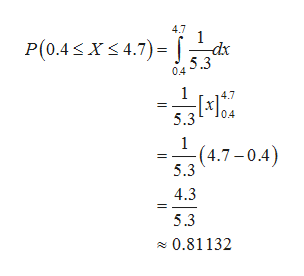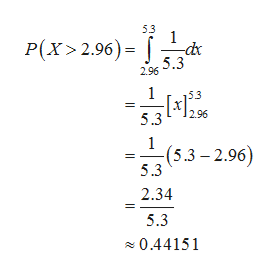# Today, the waves are crashing onto the beach every 5.3 seconds. The times from when a person arrives at the shoreline until a crashing wave is observed follows a Uniform distribution from 0 to 5.3 seconds. Round to 4 decimal places where possible.The probability that the wave will crash onto the beach between 0.4 and 4.7 seconds after the person arrives is P(0.4 < x < 4.7) = The probability that it will take longer than 2.96 seconds for the wave to crash onto the beach after the person arrives is P(x ≥≥ 2.96) = Find the maximum for the lower quartile.  seconds.

Question

Today, the waves are crashing onto the beach every 5.3 seconds. The times from when a person arrives at the shoreline until a crashing wave is observed follows a Uniform distribution from 0 to 5.3 seconds. Round to 4 decimal places where possible.

The probability that the wave will crash onto the beach between 0.4 and 4.7 seconds after the person arrives is P(0.4 < x < 4.7) =

The probability that it will take longer than 2.96 seconds for the wave to crash onto the beach after the person arrives is P(x ≥≥ 2.96) =

Find the maximum for the lower quartile.  seconds.

check_circleExpert Solution
Step 1

Let X be the random variable uniformly distributed between 0 and 5.3 seconds.

Denote X as the time from a person arrives at the shoreline until a crashing wave is observed.

Thus, the probability density function of X is:

Step 2

The probability that the wave will crash on to the beach between 0.4 and 4.7 seconds after the person arrives is calculated as follows:help_outlineImage Transcriptionclose4.7 P(0.4 X 4.7) -dx 5.3 0.4 1 0.4 5.3 1 (4.7-0.4) 5.3 4.3 5.3 0.81132 fullscreen
Step 3

The probability that it will take longer than 2.96 seconds for the wave to cr...help_outlineImage Transcriptionclose53 1 P(X>2.96)= 5.3 2.96 1 296 5.3 1 (5.3-2.96) 5.3 2.34 5.3 0.44151 fullscreen

### Want to see the full answer?

See Solution

#### Want to see this answer and more?

Solutions are written by subject experts who are available 24/7. Questions are typically answered within 1 hour*

See Solution
*Response times may vary by subject and question
Tagged in

### Other